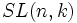# Special affine group

Let$n$ be a natural number and$k$ be a field. The special affine group or affine special linear group of degree$n$ over$k$, denoted$SA(n,k)$,$SA_n(k)$,$ASL(n,k)$, or$ASL_n(k)$, is defined as the external semidirect product of the vector space$k^n$ by the special linear group$SL(n,k)$.